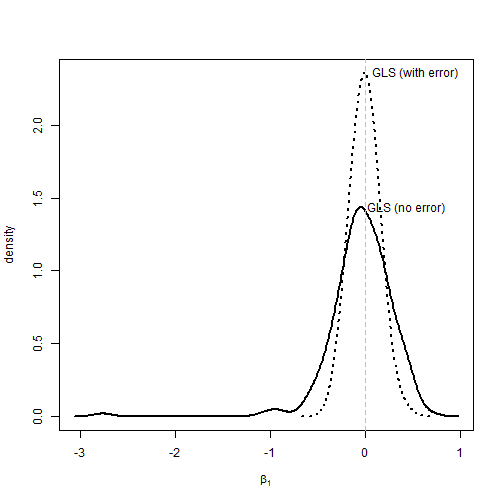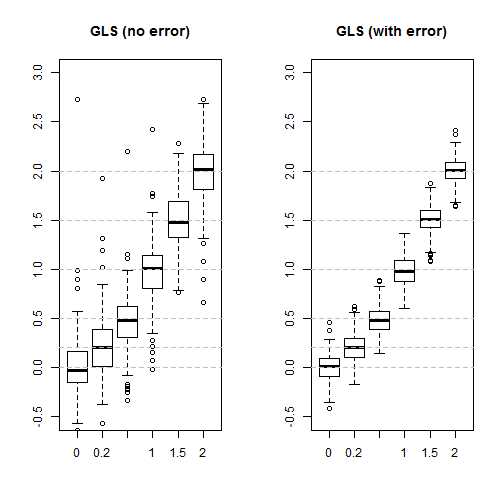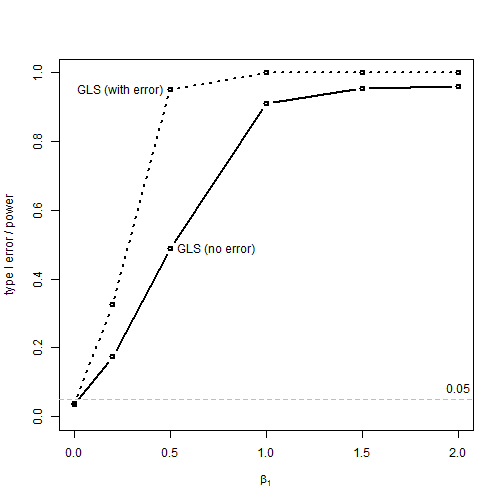## Friday, March 3, 2017

### Statistical behavior of PGLS taking account sampling error of y

Earlier today I posted on taking sampling error in y into account during phylogenetic regression or ANOVA.

In the following, I'm going to do a small investigation into type I error & power of this method. I will compare type I error & power to phylogenetic regression ignoring error in y.

First, type I error using a simple bivariate regression.

``````library(phytools)
``````
``````## Loading required package: ape
``````
``````## Loading required package: maps
``````
``````library(nlme)
packageVersion("phytools")
``````
``````##  '0.5.80'
``````
``````set.seed(1)
## simulate trees
trees<-pbtree(n=100,nsim=200)
## simulate true values of x & y
x<-lapply(trees,fastBM)
y<-lapply(trees,fastBM)
## simulate sampling variances for y
v<-lapply(trees, function(t) setNames(rexp(n=Ntip(t)),t\$tip.label))
ye<-mapply(function(y,v)
setNames(sampleFrom(xbar=y,xvar=v,n=rep(1,length(y))),
names(y)),y=y,v=v,SIMPLIFY=FALSE)
## fit PGLS ignoring sampling error in y
fitGLS<-function(t,x,y) gls(y~x,data.frame(x,y),
correlation=corBrownian(1,t),method="ML")
fits.gls<-mapply(fitGLS,trees,x,ye,SIMPLIFY=FALSE)
## now taking it into account
fitSEy<-function(t,x,y,v) pgls.SEy(y~x,data.frame(x,y),
tree=t,se=sqrt(v),method="ML")
fits.SEy<-mapply(fitSEy,trees,x,ye,v=v,SIMPLIFY=FALSE)
P.gls<-sapply(fits.gls,function(x) anova(x)\$"p-value")
mean(P.gls<=0.05) ## type I error method 1
``````
``````##  0.04
``````
``````P.sey<-sapply(fits.SEy,function(x) anova(x)\$"p-value")
mean(P.sey<=0.05) ## type I error method 2
``````
``````##  0.05
``````

So we should see straight away that there is no effect on type I error of ignoring sampling error in the estimation of species values for y. How about an effect on our estimated parameter, β1?

``````beta.gls<-sapply(fits.gls,function(x) coefficients(x))
beta.sey<-sapply(fits.SEy,function(x) coefficients(x))
d.gls<-density(beta.gls,bw=0.1)
d.sey<-density(beta.sey,bw=0.1)
plot(d.gls\$x,d.gls\$y,type="l",xlim=range(c(d.gls\$x,d.sey\$x)),
ylim=range(c(d.gls\$y,d.sey\$y)),ylab="density",
xlab=expression(beta),lwd=2)
lines(d.sey\$x,d.sey\$y,lwd=2,lty="dotted")
text(d.gls\$x[which(d.gls\$y==max(d.gls\$y))],
max(d.gls\$y),label="GLS (no error)",pos=4)
text(d.sey\$x[which(d.sey\$y==max(d.sey\$y))],
max(d.sey\$y),label="GLS (with error)",pos=4)
abline(v=0,lty="dashed",col="grey")
``````What's interesting here is that the type I error of both methods is basically identical; however the variance of the estimator that takes into count sampling error in y is much lower than the other.

Now, let's go a bit a farther & investigate the performance of each method for varying values of β1, our regression coefficient. We can do this as follows:

``````b1<-c(0,0.2,0.5,1,1.5,2)
P.gls<-P.sey<-beta.gls<-beta.sey<-matrix(NA,length(trees),length(b1),
dimnames=list(NULL,b1))
for(i in 1:length(b1)){
x<-lapply(trees,fastBM)
y<-mapply(function(t,x,b) b*x+fastBM(t),t=trees,x=x,
MoreArgs=list(b=b1[i]),SIMPLIFY=FALSE)
v<-lapply(trees, function(t) setNames(rexp(n=Ntip(t)),t\$tip.label))
ye<-mapply(function(y,v)
setNames(sampleFrom(xbar=y,xvar=v,n=rep(1,length(y))),
names(y)),y=y,v=v,SIMPLIFY=FALSE)
fitGLS<-function(t,x,y) gls(y~x,data.frame(x,y),
correlation=corBrownian(1,t),method="ML")
fits.gls<-mapply(fitGLS,trees,x,ye,SIMPLIFY=FALSE)
fitSEy<-function(t,x,y,v) pgls.SEy(y~x,data.frame(x,y),
tree=t,se=sqrt(v),method="ML")
fits.SEy<-mapply(fitSEy,trees,x,ye,v=v,SIMPLIFY=FALSE)
P.gls[,i]<-sapply(fits.gls,function(x) anova(x)\$"p-value")
P.sey[,i]<-sapply(fits.SEy,function(x) anova(x)\$"p-value")
beta.gls[,i]<-sapply(fits.gls,function(x) coefficients(x))
beta.sey[,i]<-sapply(fits.SEy,function(x) coefficients(x))
}
``````

First, we can look the mean parameter estimates of each method:

``````par(mfrow=c(1,2))
boxplot(beta.gls,ylim=c(-0.5,3),main="GLS (no error)")
abline(h=b1,col="grey",lty="dashed")
boxplot(beta.sey,ylim=c(-0.5,3),main="GLS (with error)")
abline(h=b1,col="grey",lty="dashed")
``````We can easily see that (although both methods are unbiased), variability among simulations is substantially higher when error in y is not taken into consideration.

We would expect this to affect our power to reject the null hypothesis of β1 = 1, and it does:

``````plot(b1,colMeans(P.gls<=0.05),type="b",lwd=2,xlim=c(0,2),
ylim=c(0,1),xlab=expression(beta),ylab="type I error / power")
lines(b1,colMeans(P.sey<=0.05),type="b",lwd=2,lty="dotted")
text(b1,colMeans(P.gls<=0.05),label="GLS (no error)",
pos=4)
text(b1,colMeans(P.sey<=0.05),label="GLS (with error)",
pos=2)
abline(h=0.05,lty="dashed",col="grey")
text(x=2,y=0.05,"0.05",pos=3)
``````Neat.